407 493 6601

# Algebra 2 - Normal Distributions

## Introduction - Normality of Data

• Data can be distributed in many ways. The data is arranged in a distribution to make it more organized and easier to analyze.
• How can we say that the data or scores are "normally distributed"? Again, answers may be dependent on the behavior or type of scores.
• If all measures of average (mean, median, mode) are equal, it is roughly considered as normally distributed.
• For instance, there are many real-life applications or naturally occurring phenomena with a distribution that has normal features like height, weight, test scores, the life expectancy of bulbs, crop yield in farms, etc. There are a few tall and a few small in terms of height. If there are many members in a certain population, there are numerous scores between the two extreme heights.
• If the scores are normally distributed, one good characteristic is these scores are closer to the mean and very close to each other. This is because the number of extreme low scores equals the number of extreme high scores.
• By graphing the distribution of continuous random variables, it will be noticed that the shape of the graph resembles a bell-shaped curve.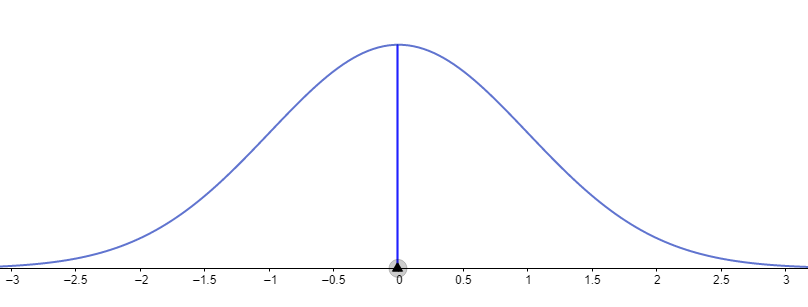• There are two tests to inspect the normality of the distribution using the SPSS (Statistical Package for Social Sciences): 1. Shapiro-Wilk Test and 2. Kolmogorov-Smirnov Test.
• Using the p-value approach when using the Shapiro-Wilk Test is the default. If the computed p-value is less than or equal to 0.05, then the distribution is approximately normally distributed. If the computed p-value is greater than 0.05, then the distribution is not normally distributed.

## Properties of the Normal Distribution

• Normal Random Variables are continuous random variables whose distribution resembles a bell-shaped curve.
• A continuous random variable is a random variable that takes on measurable characteristics. Examples are speed, time, height, weight, age, test scores, income, and the like.
• A continuous random variable whose probabilities are described by the normal distribution with mean $\mu$ and standard deviation $\sigma$ is called a normally distributed random variable or normal random variable.
• Normally distributed scores are described by a symmetrical graph of a curve called the "NORMAL CURVE." Below is the sketch of a Standard Normal Curve.• The equation (probability density function) of the normal curve is given by $f\left(x\right)=\frac{1}{\sigma \sqrt{2\mathrm{\pi }}}{e}^{-\frac{1}{2}{\left(\frac{x-\mu }{\sigma }\right)}^{2}}$ or $f\left(x\right)=\frac{1}{\sigma \sqrt{2\mathrm{\pi }}}{e}^{-\frac{1}{2}{z}^{2}}$ where x is any score in the distribution, $\sigma$ is the population standard deviation, $\mu$ is the population mean, $e$ is the Euler's number, and $\pi \approx 3.1416$.
• The distribution curve is bell-shaped.
• The curve is symmetrical about its center.
• The mean, median, and mode are at the center of the normal curve.
• The width of the curve is determined by the standard deviation of the distribution.
• The curve is asymptotic to the baseline, the x-axis.
• The total area under the normal curve is 1. The areas under the normal curve are expressed in probability values or percentages.

## Converting Normal Scores to Standard Scores

• Suppose that we have quantitative data that approximates a normal distribution with any mean $\mu$ and standard deviation $\sigma$, we compute for probabilities of normal random variables by converting them to the standard normal distribution.
• The formula that is used to convert normal random variables is the z-score equation:
• These formulas are used to standardize normal raw scores from a distribution. The term "standardized" implies that normal scores will be converted to z-scores (standard scores).

Example 1. Given a raw score of 82 from the distribution, a population mean of 85, and a standard deviation of 1.20, what is its corresponding z-score? Assume that scores are normally distributed.

Solution:

$z=\frac{X-\mu }{\sigma }$

$z=\frac{82-85}{1.20}=-2.50$

Example 2. Find the normal raw score $X$ given that its corresponding z-score is 2.5 with a population mean of 84 and a standard deviation of 1.35.

Solution:

We can use the formula $X=z\sigma +\mu$ to find the normal raw score that corresponds to $z=2.5$.

$X=z\sigma +\mu$

$X=\left(2.5\right)\left(1.35\right)+84$

$X=87.375\approx 87.38$

## Computing Probabilities using the Normal Curve

• To find the area or region under the normal curve is also to determine the probability value.
• The area under the normal curve has values between 0 and 1. The total area under the normal curve is 1.
• Important Notations:

1. $P\left(a means the probability that the z-score corresponding to a normal raw score is between the z-scores .

2. $P\left(z>a\right)$ means the probability that the z-score corresponding to a normal raw score is greater than $a$.

3. $P\left(z means the probability that the z-score corresponding to a normal raw score is less than $b$.

4. $P\left(a and $P\left(a\le z\le b\right)$ are the same in terms of computing probabilities.

Using the Standard Normal Table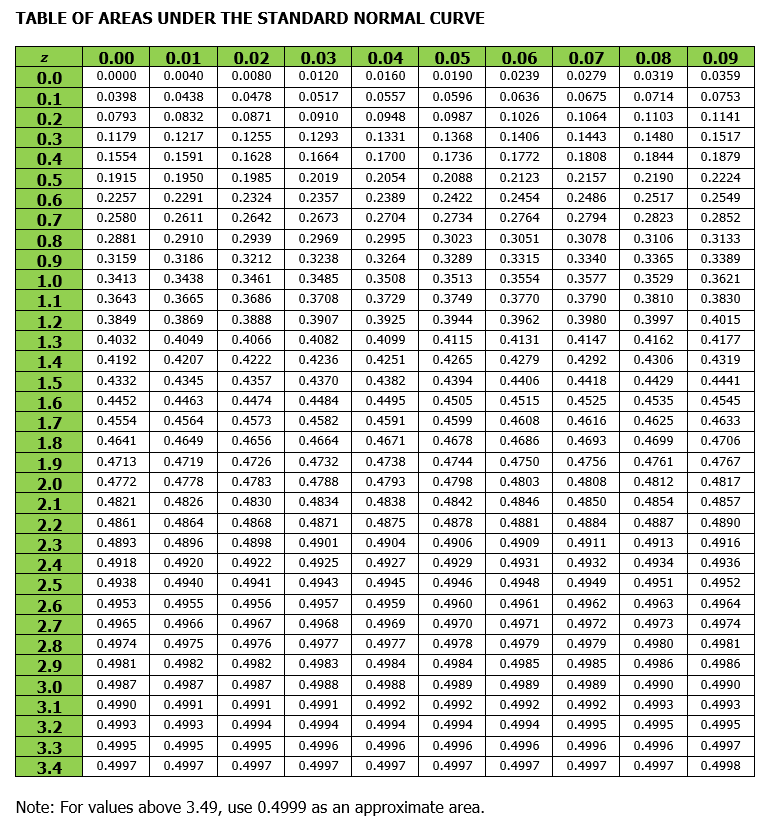The above table helps you find the probability of normal scores.

This provides the area (or probability) between $z=0$ and any value of $z$.

Observe that the row entries are the z-scores. The column entries indicate the hundredth place of a z-score.

The z-score of 1.46 in the table is the probability that the z-score lies between $z=0$ and $z=1.46$.

Using the notion of symmetry in the normal distribution, the area between $z=0$ and any value to the left is equal to the area between $z=0$ and the point equidistant to the right.

Example 1. Calculate the probability $P\left(0.

Solution:

Use a table of the area under the normal curve or the Geogebra.

The notation $P\left(0 implies we need to compute the area under the normal curve from 0 to 1.36.

Since z-scores are positive, the area is located on the right portion of the curve.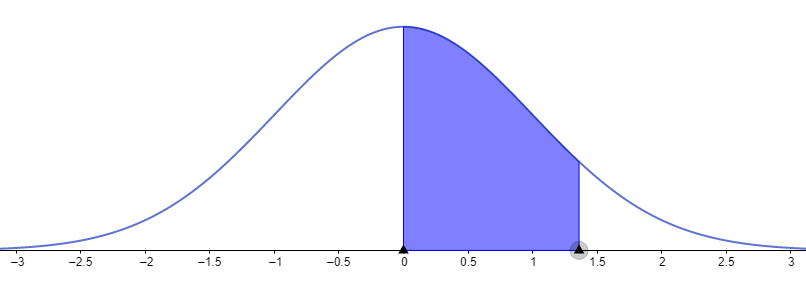Computing the area gives: $P\left(0

Example 2. Find the area between .

Solution:

The graph is shown below.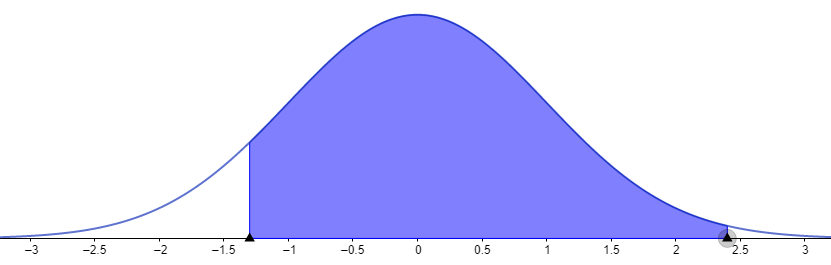Since the area includes z = 0, then we add the areas

Example 3. Compute the probability $P\left(1.25.

Solution:

The graph of $P\left(1.25 is presented below.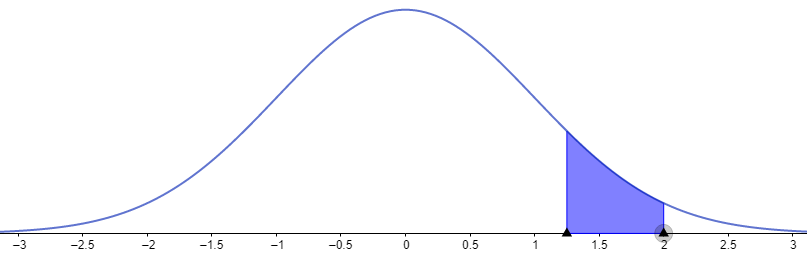To compute the probability, we need to subtract .

Example 4. What is the area under a normal curve greater than $z=1.25?$

Solution:

The graph of this area is shown below: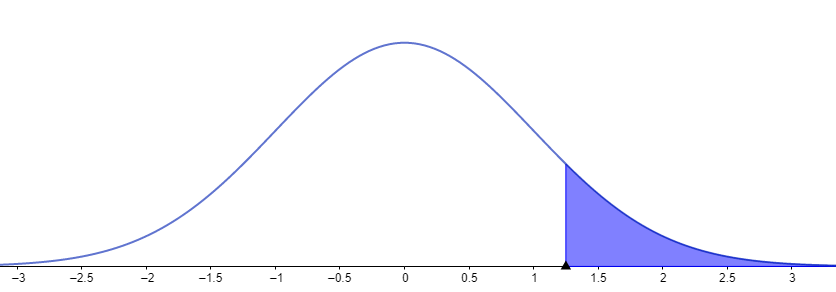$P\left(z>1.25\right)$ is computed by subtracting the area from z = 0 to z = 1.25 $P\left(0 from the half area of the normal curve, which is 0.50.

## Solved Examples

Sample Problem: In a job fair, 2500 applicants applied for contractual work. Their mean age was 32, with a standard deviation of 8 years. What is the probability that applicants have ages between 25 and 35?

Solution:

Graph: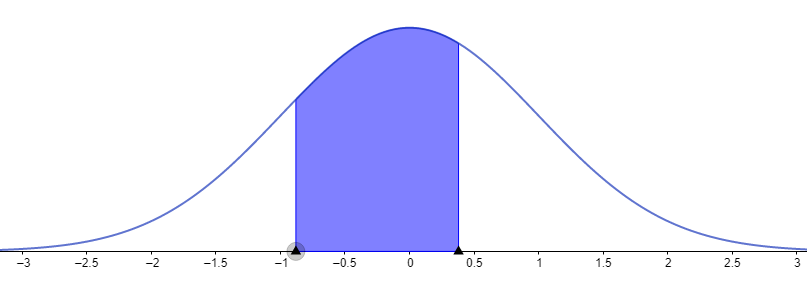To determine the probability that the applicants have ages between 25 and 35, we convert the age of 25 and age of 35 to standard scores.

The probability is written as $P\left(-0.88.

## Cheat Sheet

• In computing the probabilities, we conventionally use the table of areas under normal curve. Geogebra is also applicable for automated results and graphical presentation of the normal curve areas or probabilities.
• To compute the probability $P\left(0, we determine the area under the normal curve from This portion of the normal curve is on the right side.
• To compute the probability $P\left(-b, we find the area under the normal curve from . This portion of the normal cuve is on the left side.
• To calculate the probability $P\left(-a, we find the area under the normal curve from  and from . Add the obtained areas to get the total probability. The notation is $P\left(-a.
• When computing areas under normal curve involving a negative z-score and a positive z-score, we add the areas on the left of z = 0 and right of z = 0.
• When computing areas or probability in cases like $P\left(a wherein both z-scores are positive, we first compute the area from . Compute the area from . To find the area between z-scores , we subtract the area  from the area In symbols, we have $P\left(a.
• In cases like $P\left(z>a\right)$, we subtract the probability $P\left(0 from 0.50 (half the area of the normal curve).
• In cases like $P\left(z<-a\right)$, we subtract the probability $P\left(-a from 0.50 (half the area of the normal curve).

## Blunder Areas

• In computing areas or probabilities in normal distribution, the result is always positive. The standard scores (z-scores) have negative and positive values.
• Normal distribution cannot be applicable to discrete random variables. All normal random variables are under continuous variables.